# The Ka value for acetic acid, CH3COOH(aq), is 1.8x10^-5. Calculate the ph of a 2.80 M acetic acid solution

The Ka value for acetic acid, CH3COOH(aq), is 1.8x10^-5. Calculate the ph of a 2.80 M acetic acid solution.
PH= ?
Calculate the ph of the resulting solution when 3.00 mL of the 2.80 M acetic acid is diluted to make a 250.0 mL solution.
PH=?
Answers are not 4.6 or 3.8

Concepts and reason
Power of hydrogen ions (pH)
The pH is defined as the negative logarithmic function of the concentration of hydrogen ion in an aqueous solution.
Molar concentration:
The molar concentration is the number of moles of a solute that will dissolve in a liter of solution. It is expressed as mol/L or M (molarity).

Fundamentals
Molar concentration: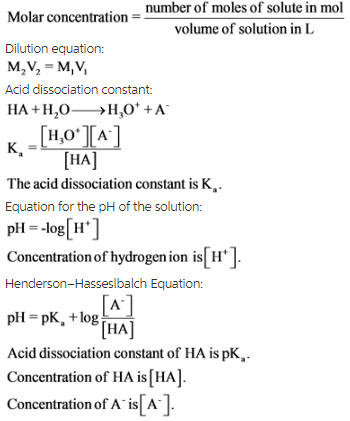An ICE table is used to find the concentrations or moles of individual reactants or products at equilibrium.
The expansion of an ICE table is as follows: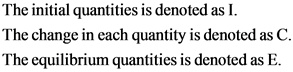All quantities are expressed in terms of concentrations or moles.

(1)
Given data:
Initial concentration of acetic acid, HA = 2.80 M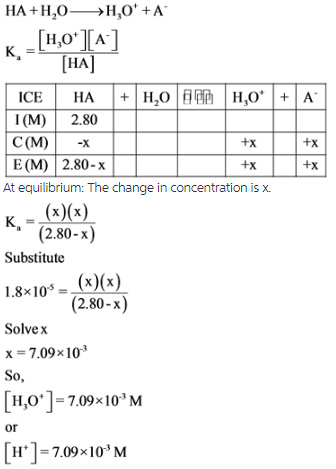An ICE table is drawn with the given quantity to find the value of change in concentration of x, which is equal to the concentration of hydrogen ion. By using x, the molar concentrations of each species are calculated with the help of acid dissociation constant expressions.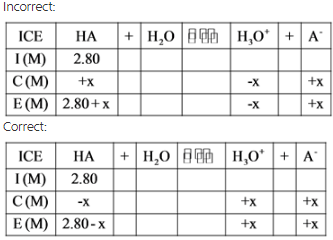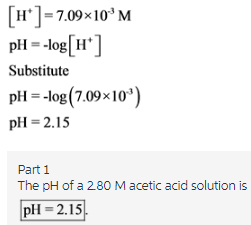The pH of the solution is calculated by substituting the value of concentration of hydrogen ion into the pH equation.
Take care while writing the pH equation. For example,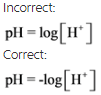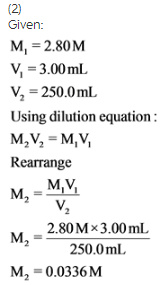The molarity of a diluted solution is obtained by substituting the values of molarity and volume of concentrated solutions and the final volume of solution.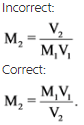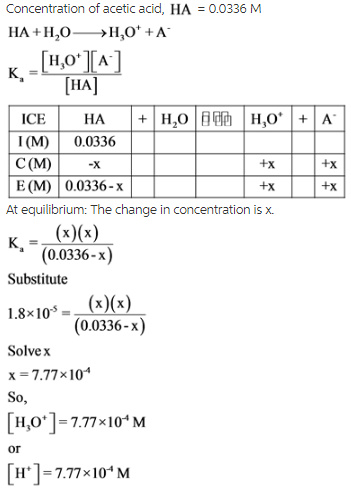An ICE table is drawn with the resulting molarity to find the value of change in concentration of x, which is equal to the concentration of the hydrogen ion. By using x, the molar concentrations of each species are calculated with the help of acid dissociation constant expressions.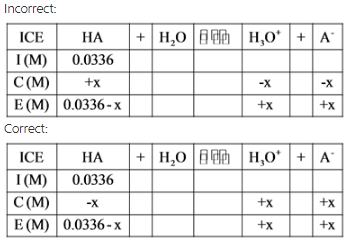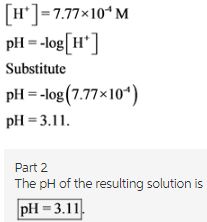The pH of the solution is calculated by substituting the value of concentration of the hydrogen ion into the pH equation.

Part 1
The pH of a 2.80 M acetic acid solution is
pH = 2.15

Part 2
The pH of the resulting solution is
pH = 3.11